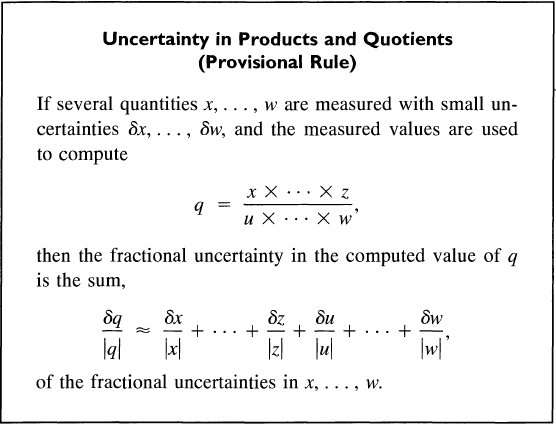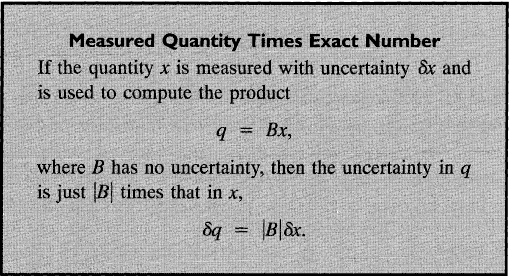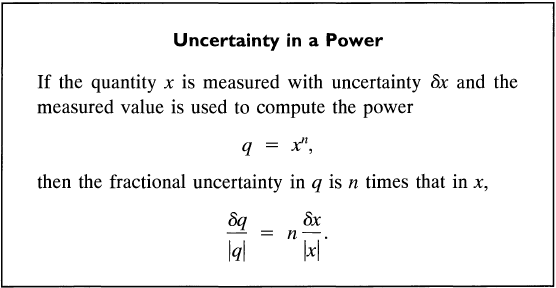# Uncertainty question

This isn't a homework question, but instead a question about an example in a book I'm reading, in prep for next semester. As such using the posting template is a bit of a miss. Hope that can be forgiven.

1. Homework Statement

I'm reading "An Introduction to Error Analysis" by John R Taylor during my spring vacation as brush up for my next semester. I encountered an example that doesn't make sense to me though. It goes through teaching 3 rules as follows:(3.8)(3.9)(3.10)

Now the example it gives is as follows (leaving out units for ease):

##t = 1.6\pm0.1##

##h = 46.2\pm0.3##

Now it calculates ##g = \frac{2*h}{t^2}## and more importantly its uncertainty as follows:

$$\frac{\delta g}{g}=\frac{\delta h}{h}+2*\frac{\delta t}{t} = 0.007+2*0.063 = 0.133$$

as justifed by 3.8 and 3.10. However here is my problem; What about 3.9?

## The Attempt at a Solution

As far as I can see, the example completely forgets about the factor 2. With 3.9 in mind, ##x = \frac{h}{t^2}## shouldn't it be:

$$\frac{\delta g}{g}=2*\left(\frac{\delta h}{h}+2*\frac{\delta t}{t}\right)$$

What am I missing?

#### Attachments

Orodruin
Staff Emeritus
Homework Helper
Gold Member
2021 Award
Honestly, you should not be using 3.8 because it essentially makes the assumption that all the errors are correlated (which may be conservative, but usually not the case). If the errors are uncorrelated, it would be more appropriate to add the relative errors in quadrature.

However, given 3.8-10: No, there should not be a factor of 2. What is given in 3.9 is ##\delta q##, not ##\delta q/q##. The factor of 2 from ##\delta q## cancels the factor of 2 from ##q##.

Edit: To expand on that, 3.9 states ##\delta q = |B| \delta x## and by definition ##|q| = |B| |x|## and therefore
$$\frac{\delta q}{|q|} = \frac{|B|\delta x}{|B| |x|} = \frac{\delta x}{|x|}.$$

Also, do not write ##\delta * x##, it is ##\delta x## which is the error in ##x##. The ##\delta## and the ##x## are part of the same symbol representing the error in ##x##.

•NicolaiTheDane
Honestly, you should not be using 3.8 because it essentially makes the assumption that all the errors are correlated (which may be conservative, but usually not the case). If the errors are uncorrelated, it would be more appropriate to add the relative errors in quadrature.

However, given 3.8-10: No, there should not be a factor of 2. What is given in 3.9 is ##\delta q##, not ##\delta q/q##. The factor of 2 from ##\delta q## cancels the factor of 2 from ##q##.

Edit: To expand on that, 3.9 states ##\delta q = |B| \delta x## and by definition ##|q| = |B| |x|## and therefore
$$\frac{\delta q}{|q|} = \frac{|B|\delta x}{|B| |x|} = \frac{\delta x}{|x|}.$$

Also, do not write ##\delta * x##, it is ##\delta x## which is the error in ##x##. The ##\delta## and the ##x## are part of the same symbol representing the error in ##x##.

Of course! Embarrassing oversight on my part. Thanks a bunch!